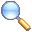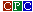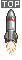High Or LowLast Update : Friday 09 April 2021 at 20 h 53

## Manual n° 1

Entry: High or Low Platform: Amstrad CPC Author: Paolo 'Neurox66' Borzini Language: Locomotive BASIC 1.1 (BASIC 1.0 is good too) Category: PUR-120 INFO: This a recreation of a popular playing card game. The objective is simple, a card is shown, the player will then choose if the next card is going to be Higher or Lower in value to the card shown. After the 41st card the deck is shuffled. I wrote the game directly on my Amstrad CPC 6128 Plus and also tested it on the following models: CPC6128 and 464 Plus. You can type the listing directly on your computer or load the "High_Low.dsk" file via M4 card or Gotek drive. Another possibility is to load the file via emulator, I tested the file via "CPCEmuMacOs" or on the following online emulators: https://www.retrovm.com http://crocods.org/web/ LOADING: Insert disk RUN"HIGHLOW.BAS" INSTRUCTIONS: Press H (High) or L (Low) for guess the value of the next card. After displaying the message whether you have guessed or not, press a key to display the next card. LISTING COMMENT: 1 Define a function for display and center the messages. Declare array for cards. 2 Valorize the array. 3..4 Shuffling the cards. 5..8 Display the cards e verify the answer. 9..10 Paint a card with a symbols. 1 DEF FNm\$(i\$)=CHR\$(31)+CHR\$(1)+CHR\$(24)+SPACE\$((80-LEN(i\$))/2)+i\$+SPACE\$((80-LEN(i\$))/2):MODE 2:DIM C(52,2):DIM C\$(13) 2 FOR I=1 to 13:READ E\$:C\$(I)=E\$:NEXT I:DATA A,2,3,4,5,6,7,8,9,10,J,Q,K:U\$=CHR\$(30)+"HIGH OR LOW By Neurox66 2021":Z\$=U\$ 3 T=0:L=0:P=0:FOR I=1 to 4:FOR II=1 to 13:P=P+1:C(P,1)=I:C(P,2)=II:NEXT II:NEXT I:PRINT FNm\$("Shuffing.."):FOR I=0 to 9 4 FOR II=1 TO 52:P=1+int(rnd*52):D=C(P,1):E=C(P,2):C(P,1)=C(II,1):C(P,2)=C(II,2):C(II,1)=D:C(II,2)=E:NEXT:NEXT:I=1 5 cls:T=T+1:PRINT Z\$:X=10:Y=205:gosub 9:if C(I+1,2) > C(I,2) then R\$="H" ELSE R\$="L":if C(I+1,2) = C(I,2) then R\$="T" 6 LOCATE 2,24:input "Is the Next Card is High or Low";Q\$:Q\$=UPPER\$(Q\$):IF Q\$ <> "H" and Q\$<> "L" goto 6 7 X=105:Y=205:I=I + 1:gosub 9:if Q\$ = R\$ or R\$="T" then print FNm\$("You're Win") else print FNm\$("You're Lose"):L=L+1 8 Z\$=U\$+" Win: "+STR\$(T-L)+" Lose: "+STR\$(L): Y\$=inkey\$:IF Y\$="" then 8 ELSE if I > 42 then 3 else 5 9 MOVE X,Y:drawr 80,0:drawr 0,120: drawr -80,0: drawr 0,-120:T\$=CHR\$(225+C(I,1)):S\$=C\$(C(I,2)) 10 LOCATE 2+X/8,32-Y/8: PRINT S\$;T\$:LOCATE 6+X/8,35-Y/8: PRINT T\$:LOCATE 8+X/8,37-Y/8: PRINT S\$;T\$:return 20 REM 1 1 1 21 REM 1 2 3 4 5 6 7 8 9 0 1 2 22 REM789012345678901234567890123456789012345678901234567890123456789012345678901234567890123456789012345678901234567890 23 REM HIGH OR LOW 25 REM BY PAOLO 'NEUROX66' Borzini 26 REM 2021 10-LINER CONTEST